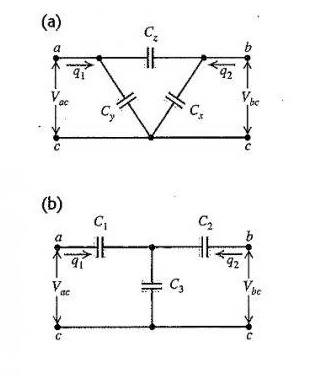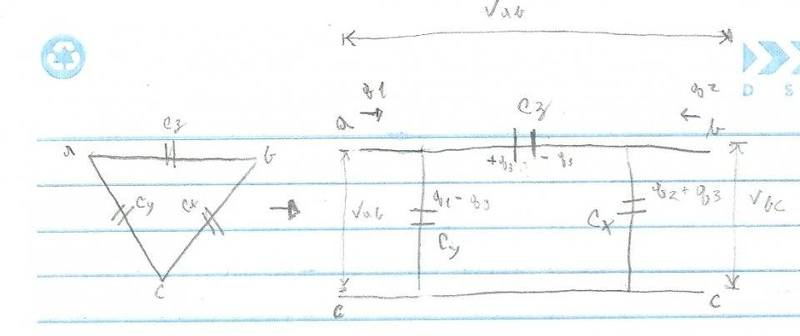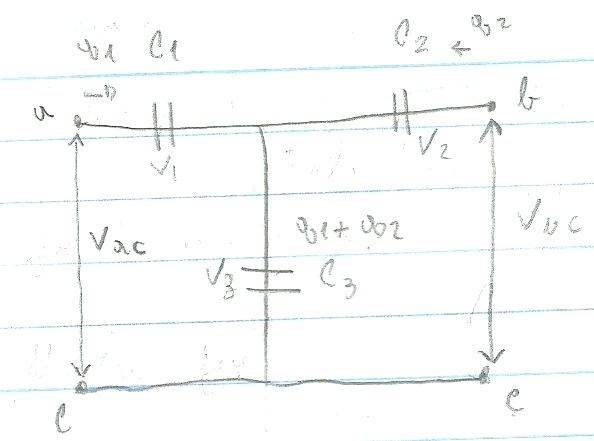# Unconventional capacitors connection

## Homework Statement

Hello everyone. The problem is related to these two capacitor connections:In the enunciate it is said that these two are equivalent and it is requested to prove that:

1. C1=(CxCy+CyCz+CzCx)/Cx
2. C2=(CxCy+CyCz+CzCx)/Cy
3. C1=(CxCy+CyCz+CzCx)/Cz

## Homework Equations

C=Q/Vab (Capacitance definition)

## The Attempt at a Solution

My problem with the solution is that I do not know how to analyze them, because of the two potentials differences.

My progress:

It is seen that the two potential differences and the two electric charges are the same in the two arrangements, so I tried to isolate Vac and Vbc in function of the electric charges: to no avail.

I noticed that in the first image, the capacitor at the top will have an electric charge merged from the two originals, that I call q3, so the left capacitor will have a charge of q1 - q3 (or q1+q3?) and the another will have a charge of q2+q3(or q2-q3?), I believe of that order do not import.

Can you give me a light on how to do this? Sorry for my poor English, I'm not proficient.

#### Attachments

•berkeman

berkeman
Mentor

## Homework Statement

Hello everyone. The problem is related to these two capacitor connections:
View attachment 111748
In the enunciate it is said that these two are equivalent and it is requested to prove that:

1. C1=(CxCy+CyCz+CzCx)/Cx
2. C2=(CxCy+CyCz+CzCx)/Cy
3. C1=(CxCy+CyCz+CzCx)/Cz

## Homework Equations

C=Q/Vab (Capacitance definition)

## The Attempt at a Solution

My problem with the solution is that I do not know how to analyze them, because of the two potentials differences.

My progress:

It is seen that the two potential differences and the two electric charges are the same in the two arrangements, so I tried to isolate Vac and Vbc in function of the electric charges: to no avail.

I noticed that in the first image, the capacitor at the top will have an electric charge merged from the two originals, that I call q3, so the left capacitor will have a charge of q1 - q3 (or q1+q3?) and the another will have a charge of q2+q3(or q2-q3?), I believe of that order do not import.

Can you give me a light on how to do this? Sorry for my poor English, I'm not proficient.
The first configuration is a π (Pi) configuration, and the second is a "T" configuration. I think the best way to work with this is to express the capacitors as impedances. Figure out the math to make the input and output impedances the same, and the transfer functions the same.

You could also do it first for resistors, since the math is a bit simpler for resistors. Maybe try it with resistors and post your work here so we can comment?haruspex
Science Advisor
Homework Helper
Gold Member
2020 Award
I tried to isolate Vac and Vbc in function of the electric charges
Not sure what you mean by that.
You understand that the question is saying that whatever the voltages applied, if you apply the same voltages to both circuits you will see the same external charge flows, right?
What equations can you write for the two circuits?

•berkeman
gneill
Mentor
Judging by the diagrams it occurs to me that this problem may originate in a course section studying 2-port networks. If that is the case there may be some benefit to investigating the so-called ABCD parameters.

I won't offer any further hints until @Drako Amorim returns to the thread to confirm.

Judging by the diagrams it occurs to me that this problem may originate in a course section studying 2-port networks. If that is the case there may be some benefit to investigating the so-called ABCD parameters.

I won't offer any further hints until @Drako Amorim returns to the thread to confirm.

This problem came from the twelfth edition Sears & Zemansky Electromagnetism, is one of the challenging problems. I think that I solved it and I will transcribe it, but I think that problem is unfair, because there's no 2-port network in the main text

gneill
Mentor
This problem came from the twelfth edition Sears & Zemansky Electromagnetism, is one of the challenging problems. I think that I solved it and I will transcribe it, but I think that problem is unfair, because there's no 2-port network in the main text
Well there are several possible approaches to solving the problem as given. The 2-port network approach occurred to me as possibly being intended and likely comparatively efficient.

Redraw the Delta circuit for a PiAnd I conclude that:

Vac = (q1 - q3)/Cy
Vbc = (q2 + q3)/Cx

Vab = q3/Cz = Vac + Vcb = Vac - Vbc

q3/Cz = (q1 - q3)/Cy - (q2 + q3)/Cx

q3 (Cy Cz + Cx Cz + Cx Cy)/(Cx Cy Cz) = q1/Cy - q2/Cx

∴q3 = Cx Cy Cz / (CyCz + CxCz + Cx Cy) [q1/Cy - q2/Cx] ≡ K (q1/Cy - q2/Cx)

∴ ( I ): Vac = q1/Cy - K q1/Cy2 + K q2/(Cx Cy)
( II ): Vbc = q2/Cx + K q1/(CyCx) - K q2/(Cx2)

In the second circuit:( III ): Vac = V1 + V3 = q1(1/C1 + 1/C3) + q2/C3

( IV ): Vbc = V2 + V3 = q1 (1/C3) + q2 (1/C2 + 1/C3)

Equalizing the coefficients:

( III ) = ( I ):

• 1/C3 = K/(Cx Cy) → C3 = (Cx Cy + Cy Cz + Cz Cx)/Cz
• 1/C1 + 1/C3 = 1/Cy - K/Cy2 → C1 = (Cx Cy + Cy Cz + Cz Cx)/Cx
( VI ) = ( II ):

• 1/C2 + 1/C3 = 1/Cx - K/Cx2 → C2 = (Cx Cy + Cy Cz + Cz Cx)/Cy
As we wanted to prove.

•berkeman and gneill
gneill
Mentor
Looks good.